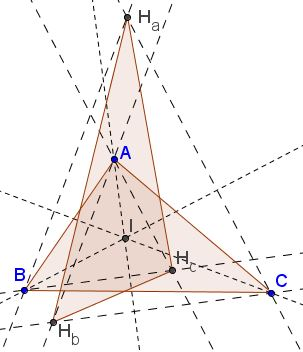# Two Related Triangles of Equal Areas

$I$ is the incenter of $\Delta ABC,$ $H_A,$ $H_b,$ $H_c$ are the orthocenters of triangles $IBC,$ $IAC,$ and $IAB,$ respectively.Prove that $[\Delta ABC]=[\Delta H_aH_bH_c]$ where $[X]$ denotes the area of figure $X.$

### Proof

$\Delta H_aBC$ is acute angled, I is its orthocenter and its angles are $\displaystyle\frac{B+C}{2},$ $\displaystyle\frac{A+B}{2},$ $\displaystyle\frac{A+C}{2},$ respectively. By the Law of Sines, $\displaystyle\frac{a}{\cos\frac{A}{2}}=2R_a,$ where $R_a$ is the circumradius of $\Delta H_aBC;$ $\displaystyle H_aI=2R_a\sin\frac{A}{2},$ implying $\displaystyle H_aI=a\tan\frac{A}{2}.$ Similarly, $\displaystyle H_bI=b\tan\frac{B}{2}.$In $\Delta IH_aH_b,$ $I=\pi -C,$ wherefrom

$\displaystyle 2[IH_aH_b]=a\tan\frac{A}{2}\cdot b\tan\frac{B}{2}\cdot\sin C=2[\Delta ABC]\tan\frac{A}{2}\cdot\tan\frac{B}{2}.$

Finally, $\displaystyle 2[\Delta H_aH_bH_c]=2[ABC]\sum_{cyc}\tan\frac{A}{2}\tan\frac{B}{2}=2[\Delta ABC].$

### Acknowledgment

The problem has been posted by Gabi Cuc at the CutTheKnotMath facebook page with credits to Marian Cucoanes. The elegant solution is by Leo Giugiuc.